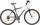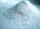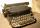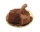Fixed expenses 2013

Since 2013, the Slovak government plans to tax more small one person businesses. Instead of fixed expences 40% will be fixed expences 40% of gross income up to 420 Eur.

Calculate what percentage of fixed expences will in 2013 pays from gross income 1569 Eur.

Result

x =  26.8 %

Solution:Leave us a comment of this math problem and its solution (i.e. if it is still somewhat unclear...):Be the first to comment!To solve this verbal math problem are needed these knowledge from mathematics:

Our percentage calculator will help you quickly calculate various typical tasks with percentages.

Next similar math problems:

1. Red diplomasHe numbers of students with honors in 2013 and 2014 are in ratio 40:49. How big is the year-on-year percentage increase?
2. Profit gainIf 5% more is gained by selling an article for Rs. 350 than by selling it for Rs. 340, the cost of the article is:
3. Highway repairThe highway repair was planned for 15 days. However, it was reduced by 30%. How many days did the repair of the highway last?
4. SummerjobThe temporary workers planted new trees. Of the total number of 500 seedlings, they managed to plant 426. How many percents did they meet the daily planting limit?
5. Percentages52 is what percent of 93?
6. Frameworks is badCalculate how many percent will increase the length of an HTML document, if any ASCII character unnecessarily encoded as hexadecimal HTML entity composed of six characters (ampersand, grid #, x, two hex digits and the semicolon). Ie. space as: &#x20;
7. Liters od milkThe cylinder-shaped container contains 80 liters of milk. Milk level is 45 cm. How much milk will in the container, if level raise to height 72 cm?
8. The ballThe ball was discounted by 10 percent and then again by 30 percent. How many percent of the original price is now?
9. PercentsHow many percents is 900 greater than the number 750?
10. PersonsPersons surveyed:100 with result: Volleyball=15% Baseball=9% Sepak Takraw=8% Pingpong=8% Basketball=60% Find the average how many like Basketball and Volleyball. Please show your solution.
11. Percentage 1052 shorts and missed 13. Calculate percentage
12. SistersSisters Zuzka Lenka and Vierka has save for a new bike. Zuzka save € 90, Lenka save € 90 and Vierka save € 82. Missing 30% gave them their mother. What is the price of the bike?
13. Bath saltsBath salt A weighs 900 grams and costs 36,40 CZK. Bath Salts B weighs 1000 grams, but costs 85 CZK. How much is a bath salt A cheaper than B one?
14. Copy typistTypist for 12 hours rewritten 15% of the manuscript. After how many hours he will done 35% of the manuscript?
15. Apples 14Maya bought 18 apples. She used 50% of the apples to make apple pies and 1/3 of the apples was mixed with other fruits to make salad. How many apples were left?
16. The salesThe sales tax rate is 4.447​% for the city and 4​% for the state. Find the total amount paid for 2 boxes of chocolates at ​\$17.96 each.
17. Percentage increaseIncrease number 400 by 3.5%